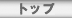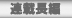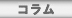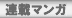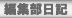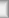# \$B\$(\$C\$A\$c\$s\$,MDG/;(;o\$rGc\$C\$F\$b\$i\$C\$?J}K!\$r65\$(\$^\$9(J

Ecchan teaches the method that had you buy an early childhood magazine

## doru

\$B!!%^%^\$K\$*6b\$,\$J\$/\$F\$b\$(\$C\$A\$c\$s\$,\$I\$&\$d\$C\$FMDG/;(;o\$r<+J,\$N\$b\$N\$K\$G\$-\$k\$+#H#O#W!!#T#OJ*8l\$G\$9!#\$A\$g\$C\$H9,\$;\$J2HB2\$J\$N\$G\$9!#(J

\$B7G:\9f(J
\$B%?%\$%H%k(J
HTML
\$BBh#1#2#39f(J
\$B\$(\$C\$A\$c\$s\$,MDG/;(;o\$rGc\$C\$F\$b\$i\$C\$?J}K!\$r65\$(\$^\$9(J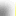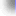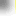\$B%H%C%W(J\$B!?(J\$BFI@ZC;JT(J\$B!?(J\$BO":\D9JT(J\$B!?(J\$B%3%i%`(J
\$B%V%C%/%l%S%e!<(J\$B!?(J\$BCx\$B!?(J\$BO":\%^%s%,(J\$B!?(JBBS\$B!?(J\$BJT=8ItF|5-(J
\$BCx:n8"(J\$B!?(J\$B%W%i%\$%P%7!<%]%j%7!<(J\$B!?(J\$B%5%\$%H%^%C%W(J